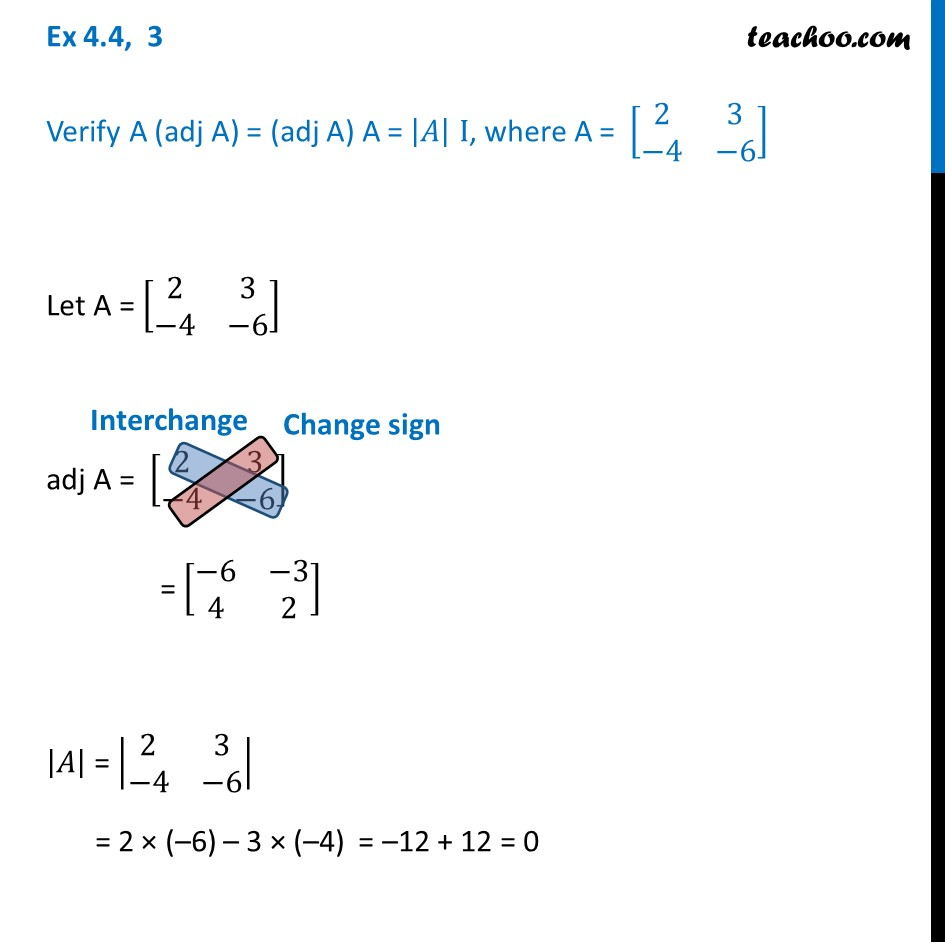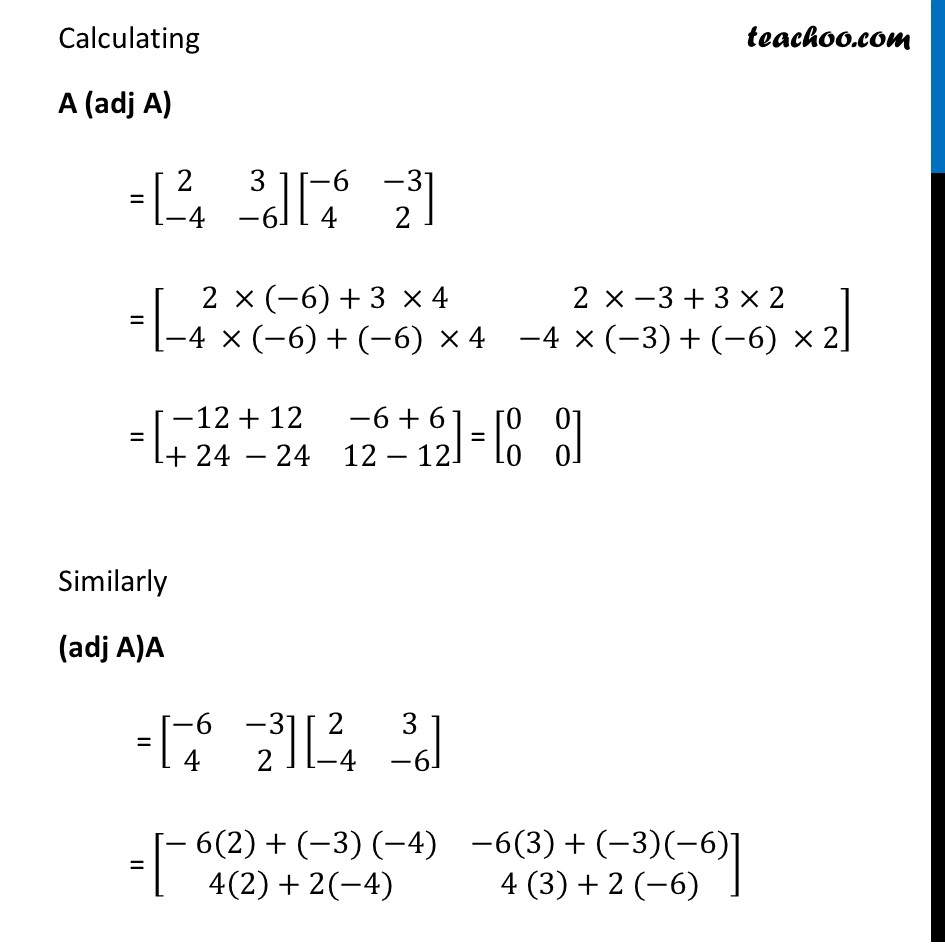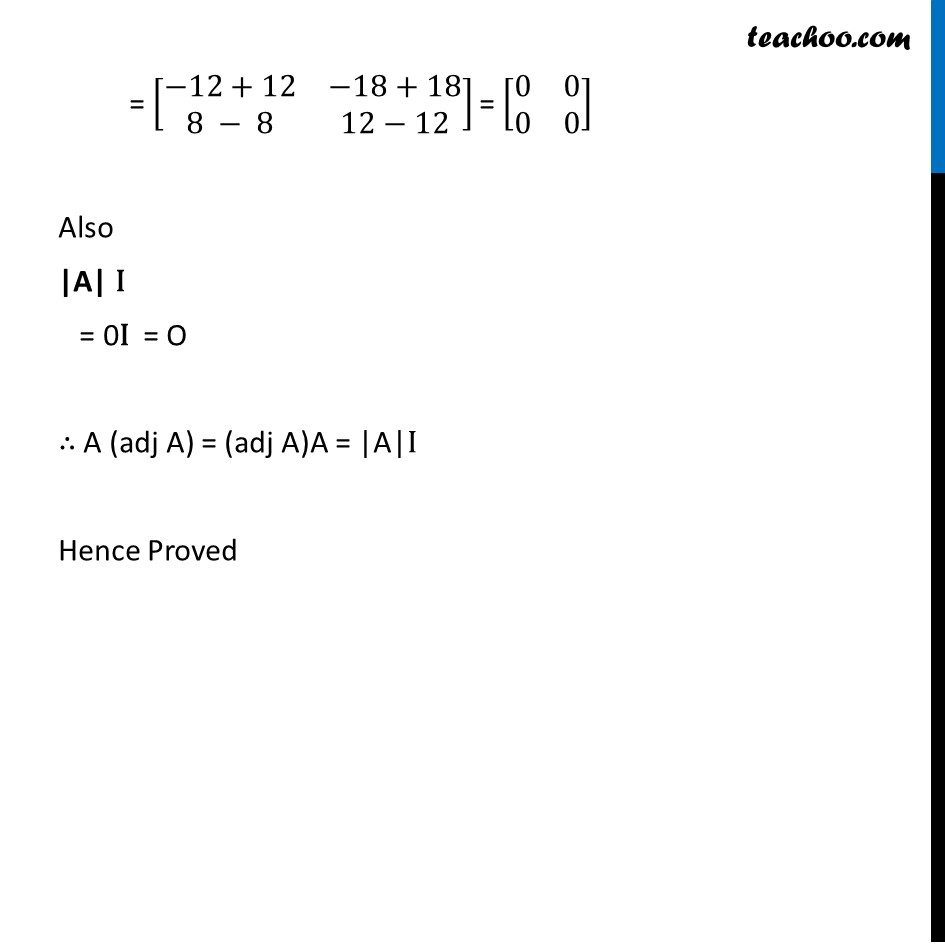Ex 4.4

Chapter 4 Class 12 Determinants
Serial order wiseLearn in your speed, with individual attention - Teachoo Maths 1-on-1 Class

### Transcript

Ex 4.4, 3 Verify A (adj A) = (adj A) A = |𝐴| I, where A = [■8(2&3@−4&−6)] Let A = [■8(2&3@−4&−6)] adj A = [■8(2&3@−4&−6)] = [■8(−6&−3@4&2)] |𝐴| = |■8(2&3@−4&−6)| = 2 × (–6) – 3 × (–4) = –12 + 12 = 0 Calculating A (adj A) = [■8(2&3@−4&−6)] [■8(−6&−3@4&2)] = [■8(2 ×(−6)+3 ×4&2 ×−3+3×2@−4 ×(−6)+(−6) ×4&−4 ×(−3)+(−6) ×2)] = [■8(−12+12&−6+6@+ 24 −24&12−12)] = [■8(0&0@0&0)] Similarly (adj A)A = [■8(−6&−3@4&2)] [■8(2&3@−4&−6)] = [■8(− 6(2)+(−3) (−4)&−6(3)+(−3)(−6)@4(2)+2(−4)&4 (3)+2 (−6))] = [■8(−12+12&−18+18@8 − 8&12−12)] = [■8(0&0@0&0)] Also |A| I = 0I = O ∴ A (adj A) = (adj A)A = |A|I Hence Proved